Elementary Algebra 2e

# 1.8The Real Numbers

Elementary Algebra 2e1.8 The Real Numbers

### Learning Objectives

By the end of this section, you will be able to:

• Simplify expressions with square roots
• Identify integers, rational numbers, irrational numbers, and real numbers
• Locate fractions on the number line
• Locate decimals on the number line

### Be Prepared 1.8

A more thorough introduction to the topics covered in this section can be found in the Prealgebra chapters, Decimals and Properties of Real Numbers.

### Simplify Expressions with Square Roots

Remember that when a number n is multiplied by itself, we write $n2n2$ and read it “n squared.” The result is called the square of n. For example,

$82read‘8squared’6464is called thesquareof8.82read‘8squared’6464is called thesquareof8.$

Similarly, 121 is the square of 11, because $112112$ is 121.

### Square of a Number

If $n2=m,n2=m,$ then m is the square of n.

### Manipulative Mathematics

Doing the Manipulative Mathematics activity “Square Numbers” will help you develop a better understanding of perfect square numbers.

Complete the following table to show the squares of the counting numbers 1 through 15.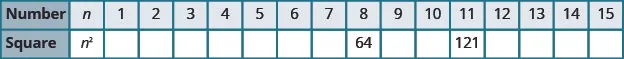The numbers in the second row are called perfect square numbers. It will be helpful to learn to recognize the perfect square numbers.

The squares of the counting numbers are positive numbers. What about the squares of negative numbers? We know that when the signs of two numbers are the same, their product is positive. So the square of any negative number is also positive.

$(−3)2=9(−8)2=64(−11)2=121(−15)2=225(−3)2=9(−8)2=64(−11)2=121(−15)2=225$

Did you notice that these squares are the same as the squares of the positive numbers?

Sometimes we will need to look at the relationship between numbers and their squares in reverse. Because $102=100,102=100,$ we say 100 is the square of 10. We also say that 10 is a square root of 100. A number whose square is $mm$ is called a square root of m.

### Square Root of a Number

If $n2=m,n2=m,$ then n is a square root of m.

Notice $(−10)2=100(−10)2=100$ also, so $−10−10$ is also a square root of 100. Therefore, both 10 and $−10−10$ are square roots of 100.

So, every positive number has two square roots—one positive and one negative. What if we only wanted the positive square root of a positive number? The radical sign, $m,m,$ denotes the positive square root. The positive square root is called the principal square root. When we use the radical sign that always means we want the principal square root.

We also use the radical sign for the square root of zero. Because $02=0,02=0,$ $0=0.0=0.$ Notice that zero has only one square root.

### Square Root Notation

$mm$ is read “the square root of m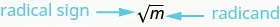If $m=n2,m=n2,$ then $m=n,m=n,$ for $n≥0.n≥0.$

The square root of m, $m,m,$ is the positive number whose square is m.

Since 10 is the principal square root of 100, we write $100=10.100=10.$ You may want to complete the following table to help you recognize square roots.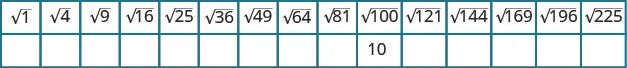### Example 1.108

Simplify: $2525$ $121.121.$

### Try It 1.215

Simplify: $3636$ $169.169.$

### Try It 1.216

Simplify: $1616$ $196.196.$

We know that every positive number has two square roots and the radical sign indicates the positive one. We write $100=10.100=10.$ If we want to find the negative square root of a number, we place a negative in front of the radical sign. For example, $−100=−10.−100=−10.$ We read $−100−100$ as “the opposite of the square root of 10.”

### Example 1.109

Simplify: $−9−9$ $−144.−144.$

### Try It 1.217

Simplify: $−4−4$ $−225.−225.$

### Try It 1.218

Simplify: $−81−81$ $−100.−100.$

### Identify Integers, Rational Numbers, Irrational Numbers, and Real Numbers

We have already described numbers as counting numbers, whole numbers, and integers. What is the difference between these types of numbers?

$Counting numbers1,2,3,4,…Whole numbers0,1,2,3,4,…Integers…−3,−2,−1,0,1,2,3,…Counting numbers1,2,3,4,…Whole numbers0,1,2,3,4,…Integers…−3,−2,−1,0,1,2,3,…$

What type of numbers would we get if we started with all the integers and then included all the fractions? The numbers we would have form the set of rational numbers. A rational number is a number that can be written as a ratio of two integers.

### Rational Number

A rational number is a number of the form $pq,pq,$ where p and q are integers and $q≠0.q≠0.$

A rational number can be written as the ratio of two integers.

All signed fractions, such as $45,−78,134,−20345,−78,134,−203$ are rational numbers. Each numerator and each denominator is an integer.

Are integers rational numbers? To decide if an integer is a rational number, we try to write it as a ratio of two integers. Each integer can be written as a ratio of integers in many ways. For example, 3 is equivalent to $31,62,93,124,155…31,62,93,124,155…$

An easy way to write an integer as a ratio of integers is to write it as a fraction with denominator one.

$3=31−8=−810=013=31−8=−810=01$

Since any integer can be written as the ratio of two integers, all integers are rational numbers! Remember that the counting numbers and the whole numbers are also integers, and so they, too, are rational.

What about decimals? Are they rational? Let’s look at a few to see if we can write each of them as the ratio of two integers.

We’ve already seen that integers are rational numbers. The integer $−8−8$ could be written as the decimal $−8.0.−8.0.$ So, clearly, some decimals are rational.

Think about the decimal 7.3. Can we write it as a ratio of two integers? Because 7.3 means $7310,7310,$ we can write it as an improper fraction, $7310.7310.$ So 7.3 is the ratio of the integers 73 and 10. It is a rational number.

In general, any decimal that ends after a number of digits (such as 7.3 or $−1.2684)−1.2684)$ is a rational number. We can use the reciprocal (or multiplicative inverse) of the place value of the last digit as the denominator when writing the decimal as a fraction.

### Example 1.110

Write as the ratio of two integers: $−27−27$ 7.31.

### Try It 1.219

Write as the ratio of two integers: $−24−24$ 3.57.

### Try It 1.220

Write as the ratio of two integers: $−19−19$ 8.41.

Let’s look at the decimal form of the numbers we know are rational.

We have seen that every integer is a rational number, since $a=a1a=a1$ for any integer, a. We can also change any integer to a decimal by adding a decimal point and a zero.

$Integer−2−10123Decimal form−2.0−1.00.01.02.03.0Integer−2−10123Decimal form−2.0−1.00.01.02.03.0$
$These decimal numbers stop.These decimal numbers stop.$

We have also seen that every fraction is a rational number. Look at the decimal form of the fractions we considered above.

$Ratio of integers45−78134−203The decimal form0.8−0.8753.25−6.666…−6.6–Ratio of integers45−78134−203The decimal form0.8−0.8753.25−6.666…−6.6–$
$These decimals either stop or repeat.These decimals either stop or repeat.$

What do these examples tell us?

Every rational number can be written both as a ratio of integers, $(pq,(pq,$ where p and q are integers and $q≠0),q≠0),$ and as a decimal that either stops or repeats.

Here are the numbers we looked at above expressed as a ratio of integers and as a decimal:

Fractions Integers
Number $4545$ $−78−78$ $134134$ $−203−203$ $−2−2$ $−1−1$ $00$ $11$ $22$ $33$
Ratio of Integers $4545$ $−78−78$ $134134$ $−203−203$ $−21−21$ $−11−11$ $0101$ $1111$ $2121$ $3131$
Decimal Form $0.80.8$ $−0.875−0.875$ $3.253.25$ $−6.6–−6.6–$ $−2.0−2.0$ $−1.0−1.0$ $0.00.0$ $1.01.0$ $2.02.0$ $3.03.0$

### Rational Number

A rational number is a number of the form $pq,pq,$ where p and q are integers and $q≠0.q≠0.$

Its decimal form stops or repeats.

Are there any decimals that do not stop or repeat? Yes!

The number $ππ$ (the Greek letter pi, pronounced “pie”), which is very important in describing circles, has a decimal form that does not stop or repeat.

$π=3.141592654...π=3.141592654...$

We can even create a decimal pattern that does not stop or repeat, such as

$2.01001000100001…2.01001000100001…$

Numbers whose decimal form does not stop or repeat cannot be written as a fraction of integers. We call these numbers irrational.

### Irrational Number

An irrational number is a number that cannot be written as the ratio of two integers.

Its decimal form does not stop and does not repeat.

Let’s summarize a method we can use to determine whether a number is rational or irrational.

### Rational or Irrational?

If the decimal form of a number

• repeats or stops, the number is rational.
• does not repeat and does not stop, the number is irrational.

### Example 1.111

Given the numbers $0.583–,0.47,3.605551275...0.583–,0.47,3.605551275...$ list the rational numbers irrational numbers.

### Try It 1.221

For the given numbers list the rational numbers irrational numbers: $0.29,0.816–,2.515115111….0.29,0.816–,2.515115111….$

### Try It 1.222

For the given numbers list the rational numbers irrational numbers: $2.63–,0.125,0.418302…2.63–,0.125,0.418302…$

### Example 1.112

For each number given, identify whether it is rational or irrational: $3636$ $44.44.$

### Try It 1.223

For each number given, identify whether it is rational or irrational: $8181$ $17.17.$

### Try It 1.224

For each number given, identify whether it is rational or irrational: $116116$ $121.121.$

We have seen that all counting numbers are whole numbers, all whole numbers are integers, and all integers are rational numbers. The irrational numbers are numbers whose decimal form does not stop and does not repeat. When we put together the rational numbers and the irrational numbers, we get the set of real numbers.

### Real Number

A real number is a number that is either rational or irrational.

All the numbers we use in elementary algebra are real numbers. Figure 1.15 illustrates how the number sets we’ve discussed in this section fit together.

Figure 1.15 This chart shows the number sets that make up the set of real numbers. Does the term “real numbers” seem strange to you? Are there any numbers that are not “real,” and, if so, what could they be?

Can we simplify $−25?−25?$ Is there a number whose square is $−25?−25?$

$()2=−25?()2=−25?$

None of the numbers that we have dealt with so far has a square that is $−25.−25.$ Why? Any positive number squared is positive. Any negative number squared is positive. So we say there is no real number equal to $−25.−25.$

The square root of a negative number is not a real number.

### Example 1.113

For each number given, identify whether it is a real number or not a real number: $−169−169$ $−64.−64.$

### Try It 1.225

For each number given, identify whether it is a real number or not a real number: $−196−196$ $−81.−81.$

### Try It 1.226

For each number given, identify whether it is a real number or not a real number: $−49−49$ $−121.−121.$

### Example 1.114

Given the numbers $−7,145,8,5,5.9,−64,−7,145,8,5,5.9,−64,$ list the whole numbers integers rational numbers irrational numbers real numbers.

### Try It 1.227

For the given numbers, list the whole numbers integers rational numbers irrational numbers real numbers: $−3,−2,0.3–,95,4,49.−3,−2,0.3–,95,4,49.$

### Try It 1.228

For the given numbers, list the whole numbers integers rational numbers irrational numbers real numbers: $−25,−38,−1,6,121,2.041975…−25,−38,−1,6,121,2.041975…$

### Locate Fractions on the Number Line

The last time we looked at the number line, it only had positive and negative integers on it. We now want to include fractions and decimals on it.

### Manipulative Mathematics

Doing the Manipulative Mathematics activity “Number Line Part 3” will help you develop a better understanding of the location of fractions on the number line.

Let’s start with fractions and locate $15,−45,3,74,−92,−5,and8315,−45,3,74,−92,−5,and83$ on the number line.

We’ll start with the whole numbers $33$ and $−5.−5.$ because they are the easiest to plot. See Figure 1.16.

The proper fractions listed are $15and−45.15and−45.$ We know the proper fraction $1515$ has value less than one and so would be located between $0 and 1.0 and 1.$ The denominator is 5, so we divide the unit from 0 to 1 into 5 equal parts $15,25,35,45.15,25,35,45.$ We plot $15.15.$ See Figure 1.16.

Similarly, $−45−45$ is between 0 and $−1.−1.$ After dividing the unit into 5 equal parts we plot $−45.−45.$ See Figure 1.16.

Finally, look at the improper fractions $74,−92,83.74,−92,83.$ These are fractions in which the numerator is greater than the denominator. Locating these points may be easier if you change each of them to a mixed number. See Figure 1.16.

$74=134−92=−41283=22374=134−92=−41283=223$

Figure 1.16 shows the number line with all the points plotted.

Figure 1.16

### Example 1.115

Locate and label the following on a number line: $4,34,−14,−3,65,−52,and73.4,34,−14,−3,65,−52,and73.$

### Try It 1.229

Locate and label the following on a number line: $−1,13,65,−74,92,5,−83.−1,13,65,−74,92,5,−83.$

### Try It 1.230

Locate and label the following on a number line: $−2,23,75,−74,72,3,−73.−2,23,75,−74,72,3,−73.$

In Example 1.116, we’ll use the inequality symbols to order fractions. In previous chapters we used the number line to order numbers.

• a < ba is less than b” when a is to the left of b on the number line
• a > ba is greater than b” when a is to the right of b on the number line

As we move from left to right on a number line, the values increase.

### Example 1.116

Order each of the following pairs of numbers, using < or >. It may be helpful to refer Figure 1.17.

$−23___−1−23___−1$ $−312___−3−312___−3$ $−34___−14−34___−14$ $−2___−83−2___−83$

Figure 1.17

### Try It 1.231

Order each of the following pairs of numbers, using < or >:

$−13___−1−13___−1$ $−112___−2−112___−2$ $−23___−13−23___−13$ $−3___−73.−3___−73.$

### Try It 1.232

Order each of the following pairs of numbers, using < or >:

$−1___−23−1___−23$ $−214___−2−214___−2$ $−35___−45−35___−45$ $−4___−103.−4___−103.$

### Locate Decimals on the Number Line

Since decimals are forms of fractions, locating decimals on the number line is similar to locating fractions on the number line.

### Example 1.117

Locate 0.4 on the number line.

### Try It 1.233

Locate on the number line: 0.6.

### Try It 1.234

Locate on the number line: 0.9.

### Example 1.118

Locate $−0.74−0.74$ on the number line.

### Try It 1.235

Locate on the number line: $−0.6.−0.6.$

### Try It 1.236

Locate on the number line: $−0.7.−0.7.$

Which is larger, 0.04 or 0.40? If you think of this as money, you know that $0.40 (forty cents) is greater than$0.04 (four cents). So,

$0.40>0.040.40>0.04$

Again, we can use the number line to order numbers.

• a < ba is less than b” when a is to the left of b on the number line
• a > ba is greater than b” when a is to the right of b on the number line

Where are 0.04 and 0.40 located on the number line? See Figure 1.20.

Figure 1.20

We see that 0.40 is to the right of 0.04 on the number line. This is another way to demonstrate that 0.40 > 0.04.

How does 0.31 compare to 0.308? This doesn’t translate into money to make it easy to compare. But if we convert 0.31 and 0.308 into fractions, we can tell which is larger.

 0.31 0.308 Convert to fractions. $3110031100$ $30810003081000$ We need a common denominator to compare them.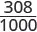$31010003101000$ $30810003081000$

Because 310 > 308, we know that $3101000>3081000.3101000>3081000.$ Therefore, 0.31 > 0.308.

Notice what we did in converting 0.31 to a fraction—we started with the fraction $3110031100$ and ended with the equivalent fraction $3101000.3101000.$ Converting $31010003101000$ back to a decimal gives 0.310. So 0.31 is equivalent to 0.310. Writing zeros at the end of a decimal does not change its value!

$31100=3101000and0.31=0.31031100=3101000and0.31=0.310$

We say 0.31 and 0.310 are equivalent decimals.

### Equivalent Decimals

Two decimals are equivalent if they convert to equivalent fractions.

We use equivalent decimals when we order decimals.

The steps we take to order decimals are summarized here.

### How To

#### Order Decimals.

1. Step 1. Write the numbers one under the other, lining up the decimal points.
2. Step 2. Check to see if both numbers have the same number of digits. If not, write zeros at the end of the one with fewer digits to make them match.
3. Step 3. Compare the numbers as if they were whole numbers.
4. Step 4. Order the numbers using the appropriate inequality sign.

### Example 1.119

Order $0.64___0.60.64___0.6$ using $<<$ or $>.>.$

### Try It 1.237

Order each of the following pairs of numbers, using $:0.42___0.4.:0.42___0.4.$

### Try It 1.238

Order each of the following pairs of numbers, using $:0.18___0.1.:0.18___0.1.$

### Example 1.120

Order $0.83___0.8030.83___0.803$ using $<<$ or $>.>.$

### Try It 1.239

Order the following pair of numbers, using $:0.76___0.706.:0.76___0.706.$

### Try It 1.240

Order the following pair of numbers, using $:0.305___0.35.:0.305___0.35.$

When we order negative decimals, it is important to remember how to order negative integers. Recall that larger numbers are to the right on the number line. For example, because $−2−2$ lies to the right of $−3−3$ on the number line, we know that $−2>−3.−2>−3.$ Similarly, smaller numbers lie to the left on the number line. For example, because $−9−9$ lies to the left of $−6−6$ on the number line, we know that $−9<−6.−9<−6.$ See Figure 1.21.

Figure 1.21

If we zoomed in on the interval between 0 and $−1,−1,$ as shown in Example 1.121, we would see in the same way that $−0.2>−0.3and−0.9<−0.6.−0.2>−0.3and−0.9<−0.6.$

### Example 1.121

Use $<<$ or $>>$ to order $−0.1___−0.8.−0.1___−0.8.$

### Try It 1.241

Order the following pair of numbers, using < or >: $−0.3___−0.5.−0.3___−0.5.$

### Try It 1.242

Order the following pair of numbers, using < or >: $−0.6___−0.7.−0.6___−0.7.$

### Section 1.8 Exercises

#### Practice Makes Perfect

Simplify Expressions with Square Roots

In the following exercises, simplify.

659.

$36 36$

660.

$4 4$

661.

$64 64$

662.

$169 169$

663.

$9 9$

664.

$16 16$

665.

$100 100$

666.

$144 144$

667.

$− 4 − 4$

668.

$− 100 − 100$

669.

$− 1 − 1$

670.

$− 121 − 121$

Identify Integers, Rational Numbers, Irrational Numbers, and Real Numbers

In the following exercises, write as the ratio of two integers.

671.

5 3.19

672.

8 1.61

673.

$−12−12$ 9.279

674.

$−16−16$ 4.399

In the following exercises, list the rational numbers, irrational numbers

675.

$0.75 , 0.22 3 – , 1.39174 … 0.75 , 0.22 3 – , 1.39174 …$

676.

$0.36 , 0.94729 … , 2.52 8 – 0.36 , 0.94729 … , 2.52 8 –$

677.

$0.4 5 – , 1.919293 … , 3.59 0.4 5 – , 1.919293 … , 3.59$

678.

$0.1 3 – , 0.42982 … , 1.875 0.1 3 – , 0.42982 … , 1.875$

In the following exercises, identify whether each number is rational or irrational.

679.

$2525$ $3030$

680.

$4444$ $4949$

681.

$164164$ $169169$

682.

$225225$ $216216$

In the following exercises, identify whether each number is a real number or not a real number.

683.

$−81−81$ $−121−121$

684.

$−64−64$ $−9−9$

685.

$−36−36$ $−144−144$

686.

$−49−49$ $−144−144$

In the following exercises, list the whole numbers, integers, rational numbers, irrational numbers, real numbers for each set of numbers.

687.

$−8 , 0 , 1.95286 … , 12 5 , 36 , 9 −8 , 0 , 1.95286 … , 12 5 , 36 , 9$

688.

$−9 , −3 4 9 , − 9 , 0.40 9 – , 11 6 , 7 −9 , −3 4 9 , − 9 , 0.40 9 – , 11 6 , 7$

689.

$− 100 , −7 , − 8 3 , −1 , 0.77 , 3 1 4 − 100 , −7 , − 8 3 , −1 , 0.77 , 3 1 4$

690.

$−6 , − 5 2 , 0 , 0. 714285 ——— , 2 1 5 , 14 −6 , − 5 2 , 0 , 0. 714285 ——— , 2 1 5 , 14$

Locate Fractions on the Number Line

In the following exercises, locate the numbers on a number line.

691.

$3 4 , 8 5 , 10 3 3 4 , 8 5 , 10 3$

692.

$1 4 , 9 5 , 11 3 1 4 , 9 5 , 11 3$

693.

$3 10 , 7 2 , 11 6 , 4 3 10 , 7 2 , 11 6 , 4$

694.

$7 10 , 5 2 , 13 8 , 3 7 10 , 5 2 , 13 8 , 3$

695.

$2 5 , − 2 5 2 5 , − 2 5$

696.

$3 4 , − 3 4 3 4 , − 3 4$

697.

$3 4 , − 3 4 , 1 2 3 , −1 2 3 , 5 2 , − 5 2 3 4 , − 3 4 , 1 2 3 , −1 2 3 , 5 2 , − 5 2$

698.

$2 5 , − 2 5 , 1 3 4 , −1 3 4 , 8 3 , − 8 3 2 5 , − 2 5 , 1 3 4 , −1 3 4 , 8 3 , − 8 3$

In the following exercises, order each of the pairs of numbers, using < or >.

699.

$−1 ___ − 1 4 −1 ___ − 1 4$

700.

$−1 ___ − 1 3 −1 ___ − 1 3$

701.

$−2 1 2 ___ −3 −2 1 2 ___ −3$

702.

$−1 3 4 ___ −2 −1 3 4 ___ −2$

703.

$− 5 12 ___ − 7 12 − 5 12 ___ − 7 12$

704.

$− 9 10 ___ − 3 10 − 9 10 ___ − 3 10$

705.

$−3 ___ − 13 5 −3 ___ − 13 5$

706.

$−4 ___ − 23 6 −4 ___ − 23 6$

Locate Decimals on the Number Line In the following exercises, locate the number on the number line.

707.

0.8

708.

$−0.9 −0.9$

709.

$−1.6 −1.6$

710.

3.1

In the following exercises, order each pair of numbers, using < or >.

711.

$0.37 ___ 0.63 0.37 ___ 0.63$

712.

$0.86 ___ 0.69 0.86 ___ 0.69$

713.

$0.91 ___ 0.901 0.91 ___ 0.901$

714.

$0.415 ___ 0.41 0.415 ___ 0.41$

715.

$−0.5 ___ −0.3 −0.5 ___ −0.3$

716.

$−0.1 ___ −0.4 −0.1 ___ −0.4$

717.

$−0.62 ___ −0.619 −0.62 ___ −0.619$

718.

$−7.31 ___ −7.3 −7.31 ___ −7.3$

#### Everyday Math

719.

Field trip All the 5th graders at Lincoln Elementary School will go on a field trip to the science museum. Counting all the children, teachers, and chaperones, there will be 147 people. Each bus holds 44 people.

How many busses will be needed?
Why must the answer be a whole number?
Why shouldn’t you round the answer the usual way, by choosing the whole number closest to the exact answer?

720.

Child care Serena wants to open a licensed child care center. Her state requires there be no more than 12 children for each teacher. She would like her child care center to serve 40 children.

How many teachers will be needed? Why must the answer be a whole number? Why shouldn’t you round the answer the usual way, by choosing the whole number closest to the exact answer?

#### Writing Exercises

721.

In your own words, explain the difference between a rational number and an irrational number.

722.

Explain how the sets of numbers (counting, whole, integer, rational, irrationals, reals) are related to each other.

#### Self Check

After completing the exercises, use this checklist to evaluate your mastery of the objective of this section.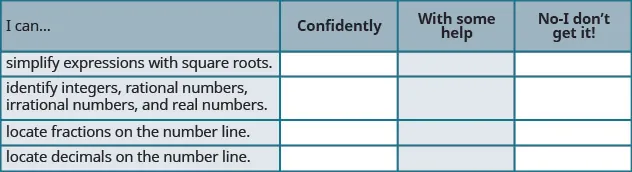On a scale of $1−10,1−10,$ how would you rate your mastery of this section in light of your responses on the checklist? How can you improve this?

Order a print copy

As an Amazon Associate we earn from qualifying purchases.